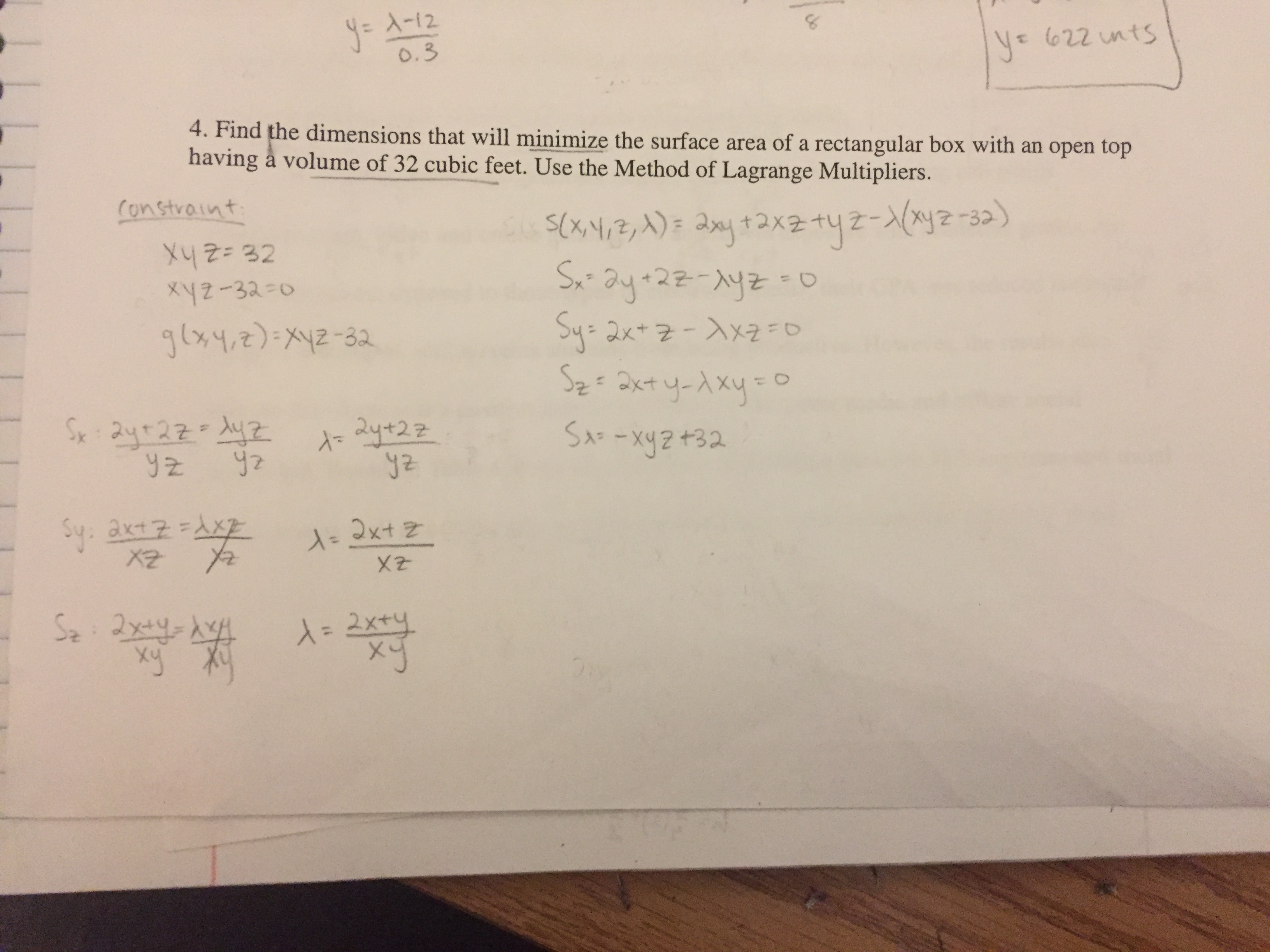# yeo.34. Find the dimensions that will minimize the surface area of a rectangular box with an open tophaving à volume of 32 cubic feet. Use the Method of Lagrange Multipliers.x2-33 o-32X2xy

Question
61 viewshelp_outlineImage Transcriptioncloseye o.3 4. Find the dimensions that will minimize the surface area of a rectangular box with an open top having à volume of 32 cubic feet. Use the Method of Lagrange Multipliers. x2-33 o -32 X2 xy fullscreen
check_circle

Step 1

Let x be the height , y and z be the dimensions of base of rectangular box, then volume is length*breadth *height

and surface area is shown on board.

Step 2

Now, consider the function F as shown on board, we will partial derivative of F with respect to the variablex, y, z and lagrange multiplier and set them equal to zero.

Step 3

On solving first three equations simultaneou...

### Want to see the full answer?

See Solution

#### Want to see this answer and more?

Solutions are written by subject experts who are available 24/7. Questions are typically answered within 1 hour.*

See Solution
*Response times may vary by subject and question.
Tagged in

### Derivative